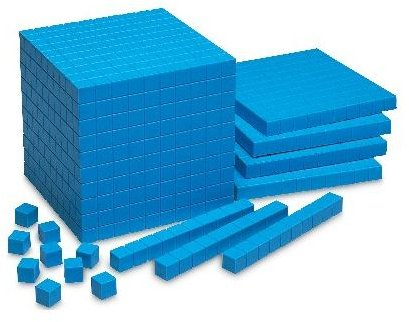# Strategies for Borrowing or How to Carry-Over on Subtraction

## Starting With the Basics

How to carry-over on subtraction, or some people refer to it as borrowing, can be confusing to young students. They know how to subtract 15-9 and get the correct answer. But when the problem looks like this 65-29, it becomes more difficult. Many students know you cannot have 5 items and take 9 away without getting a negative number. However, when they are working an entire page of subtraction problems, they may decide to do 9 – 5 instead of using the carry-over or borrow strategy. So, what do you do?

First, start with the basics of how to carry-over on subtraction by teaching students that you always subtract top to bottom. Because we teach them that with addition, it doesn’t matter which way you add the numbers (5+6 or 6+5), they may believe that same rule is true with subtraction. Do several easy problems with students–maybe have students work problems on the chalkboard–to show that with subtraction, you start with the top number and subtract the bottom number.

You can get out manipulatives for each student and ask them to put 5 blocks on their desk. Now, ask them to take 9 away. They will easily see that this is impossible.

Before students learn how to carry-over on subtraction, they need to understand these basic subtraction rules–it doesn’t hurt for any student having difficulty to review these during one class period.

## Carry-Over or Borrowing Teaching IdeasMany times, we teach students the process of working a math problem–but not the "why" behind it. If students don’t understand why and how to carry-over on subtraction, then they may have difficulty with these problems.

One way teachers show students why borrowing works is with base-10 blocks. If students are working this problem: 33-17=?, they can use base-10 blocks to understand why borrowing works. This teaching strategy is especially helpful for tactile and visual learners. Students take 3 tens bars and 3 ones cubes and put these at the top of their desks. The problem states that they need to take 7 ones cubes away–but they only have 3, so what can they do? Borrow from the tens. They learn to exchange one tens block for 10 ones cubes. Now they have 13 ones cubes, and they can take 7 away. Once students work the problems with base-10 blocks and understand what it means to carry-over on subtraction, then teachers can show them how to work the problems on paper.

Other students will respond better to a story about how to carry-over on subtraction. With the same problem as above, teachers tell students that 33 wants to take away 7 ones, but she doesn’t have 7 ones to give–what can she do? She can borrow some ones from her neighbor the tens place–just like your mom might have to borrow sugar from a neighbor to bake a cake. When 33 borrows 10 ones from the tens place, the tens won’t have as many left–they’ll only have 2 now and the ones will have 13. Just like the neighbor won’t have as much sugar if Mom takes some from her. A story like this will work for some students because they understand what "borrow" means and can remember stories well.

The important thing to remember when teaching how to carry-over on subtraction is that different methods and strategies will work for different learners.